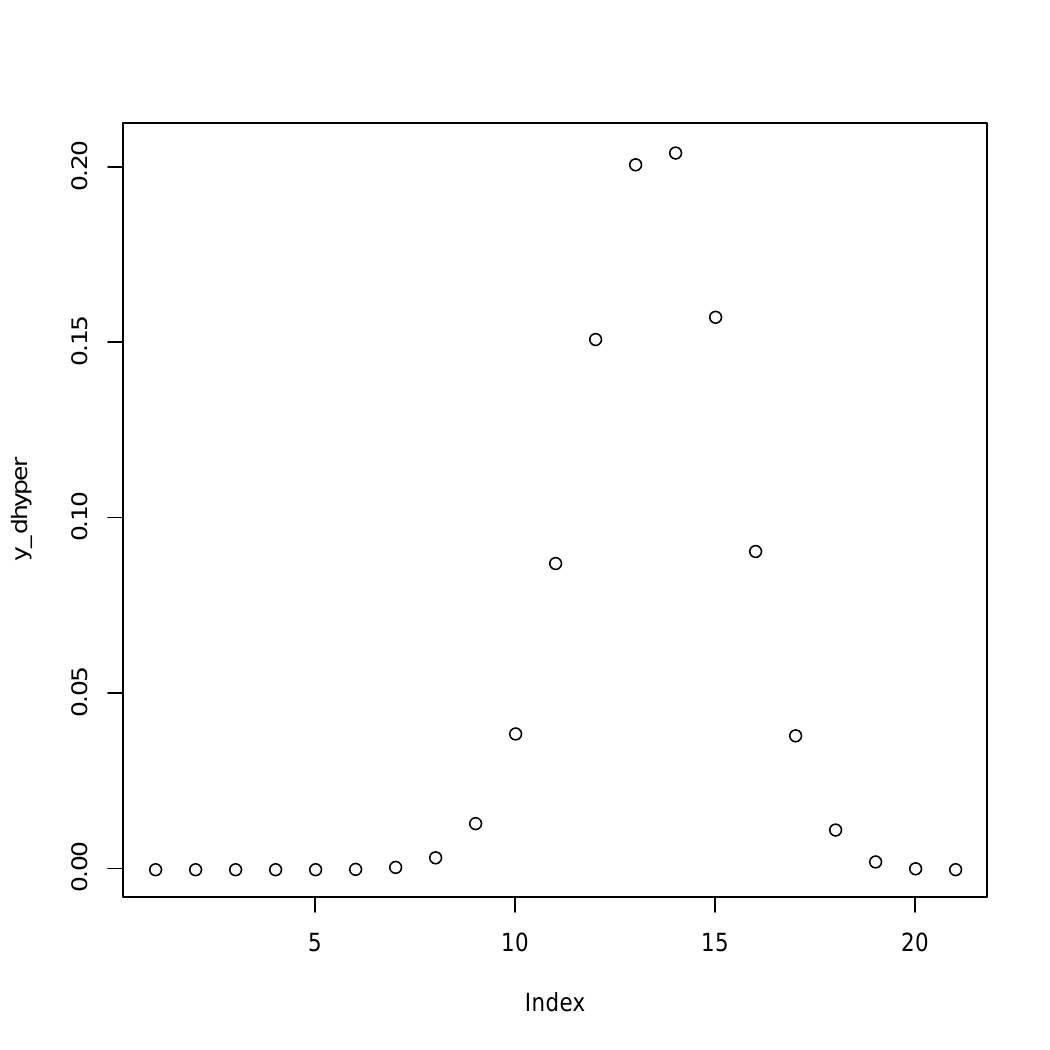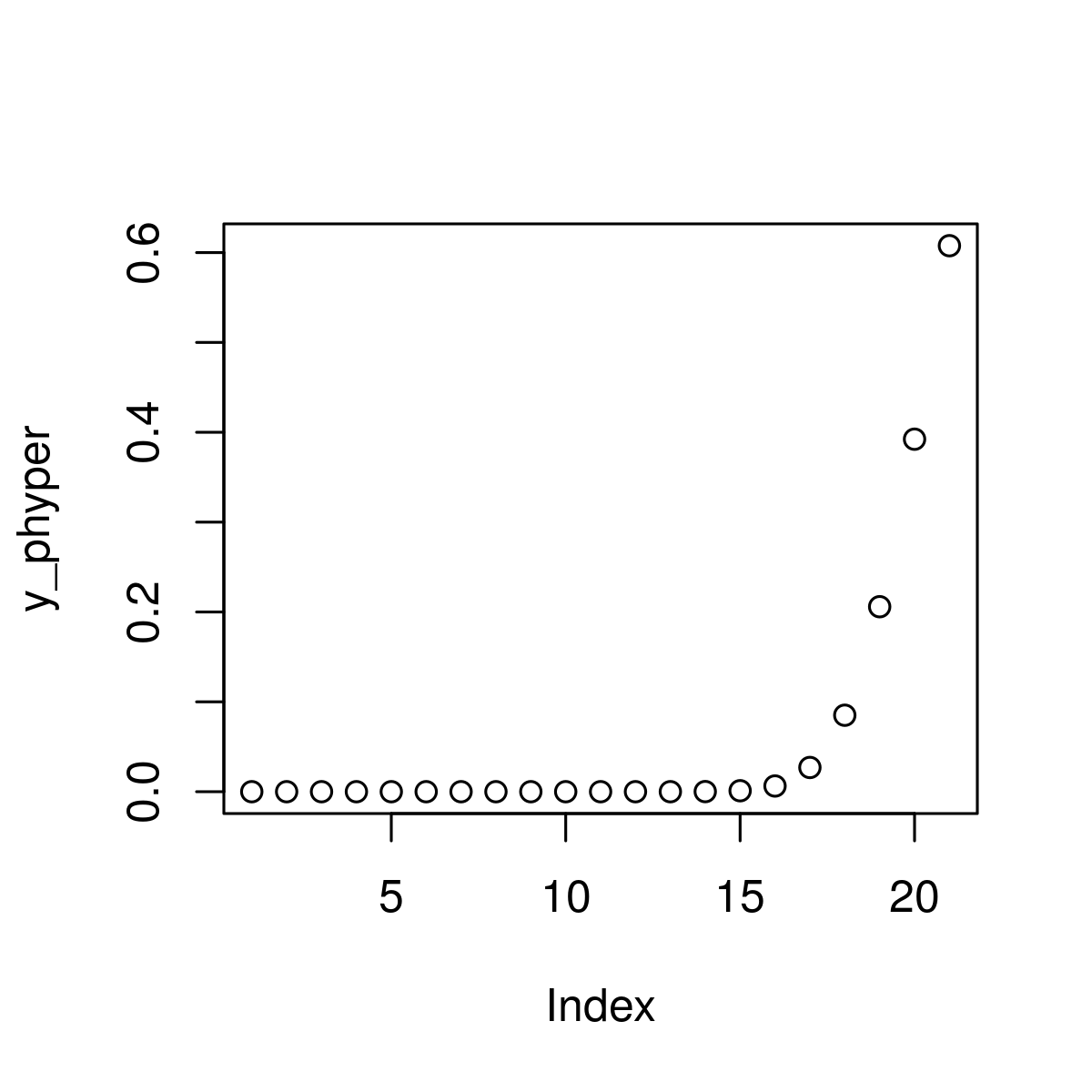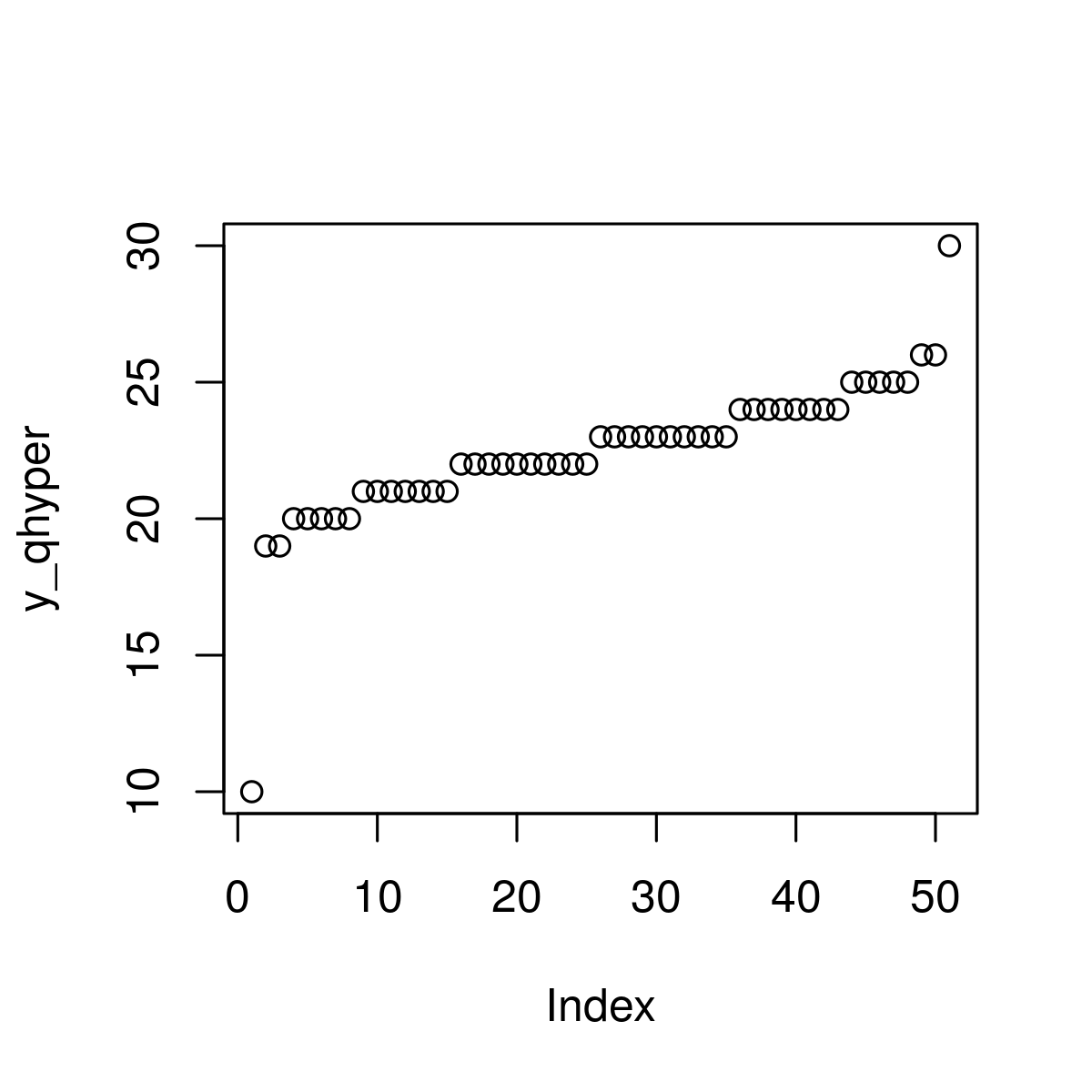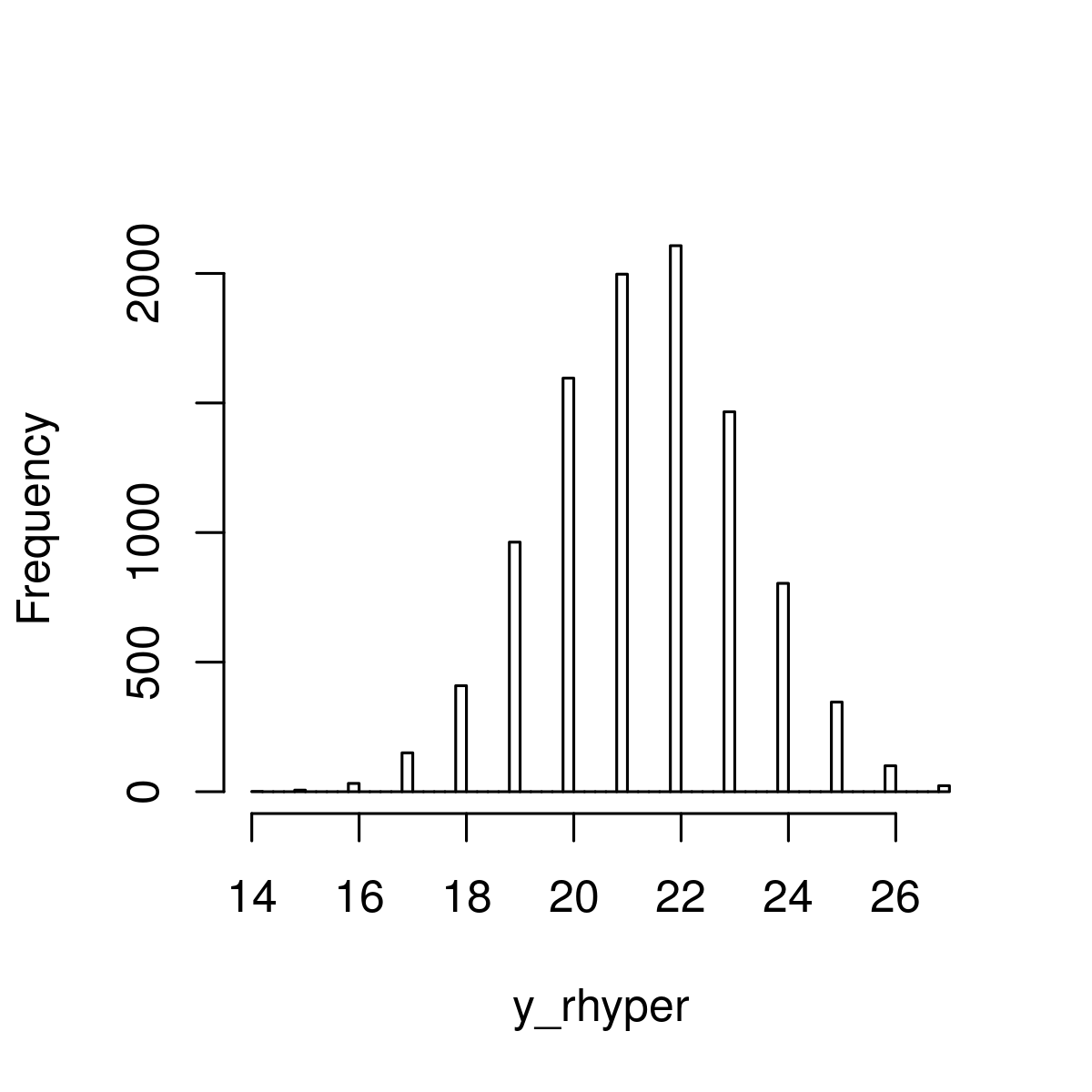# Hypergeometric Distribution in R Programming

Hypergeometric Distribution in R Language is defined as a method that is used to calculate probabilities when sampling without replacement is to be done in order to get the density value.

In R, there are 4 built-in functions to generate Hypergeometric Distribution:

• dhyper()
`dhyper(x, m, n, k)`
• phyper()
`phyper(x, m, n, k)`
• qhyper()
`qhyper(x, m, n, k)`
• rhyper()
`rhyper(N, m, n, k)`

where,

x: represents the data set of values
m: size of the population
n: number of samples drawn
k: number of items in the population
N: hypergeometrically distributed values

#### Functions To Generate Hypergeometric Distribution

##### dhyper() Function

It is defined as Hypergeometric Density Distribution used in order to get the density value.

Syntax:

```dhyper(x_dhyper, m, n, k)
```

Example 1:

 `  `  `# Specify x-values for dhyper function ` `x_dhyper <- ``seq``(0, 22, by = 1.2) ` ` `  `# Apply dhyper function ` `y_dhyper <- ``dhyper``(x_dhyper, m = 45, n = 30, k = 20)    ` `  `  `# Plot dhyper values ` `plot``(y_dhyper)  `

Output:##### phyper() Function

Hypergeometric Cumulative Distribution Function used estimating the number of faults initially resident in a program at the beginning of the test or debugging process based on the hypergeometric distribution and calculate each value in x using the corresponding values.

Syntax:

`phyper(x, m, n, k)`

Example 1:

 `# Specify x-values for phyper function ` `x_phyper <- ``seq``(0, 22, by = 1)     ` `   `  `# Apply phyper function ` `y_phyper <- ``phyper``(x_phyper, m = 40, n = 20, k = 31)   ` ` `  `# Plot phyper values ` `plot``(y_phyper)   `

Output:##### qhyper() Function

It is basically Hypergeometric Quantile Function used to specify a sequence of probabilities between 0 and 1.

Syntax:

`qhyper(x, m, n, k)`

Example 1:

 `# Specify x-values for qhyper function ` `x_qhyper <- ``seq``(0, 1, by = 0.02)         ` ` `  `# Apply qhyper function ` `y_qhyper <- ``qhyper``(x_qhyper, m = 49, n = 18, k = 30)     ` ` `  `# Plot qhyper values ` `plot``(y_qhyper) `

Output:##### rhyper() Function

It generally refers to generating random numbers function by specifying a seed and sample size.

Syntax:

`rhyper(x, m, n, k)`

Example 1:

 `# Set seed for reproducibility ` `# Specify sample size ` `set.seed``(400)                                  ` `N <- 10000                                       ` ` `  `# Draw N hypergeometrically distributed values ` `y_rhyper <- ``rhyper``(N, m = 50, n = 20, k = 30)  ` `y_rhyper          ` ` `  `# Plot of randomly drawn hyper density ` `hist``(y_rhyper,                                           ` `     ``breaks = 50, ` `     ``main = ``""``) `

Output :My Personal Notes arrow_drop_upCheck out this Author's contributed articles.

If you like GeeksforGeeks and would like to contribute, you can also write an article using contribute.geeksforgeeks.org or mail your article to contribute@geeksforgeeks.org. See your article appearing on the GeeksforGeeks main page and help other Geeks.

Please Improve this article if you find anything incorrect by clicking on the "Improve Article" button below.

Article Tags :

Be the First to upvote.

Please write to us at contribute@geeksforgeeks.org to report any issue with the above content.